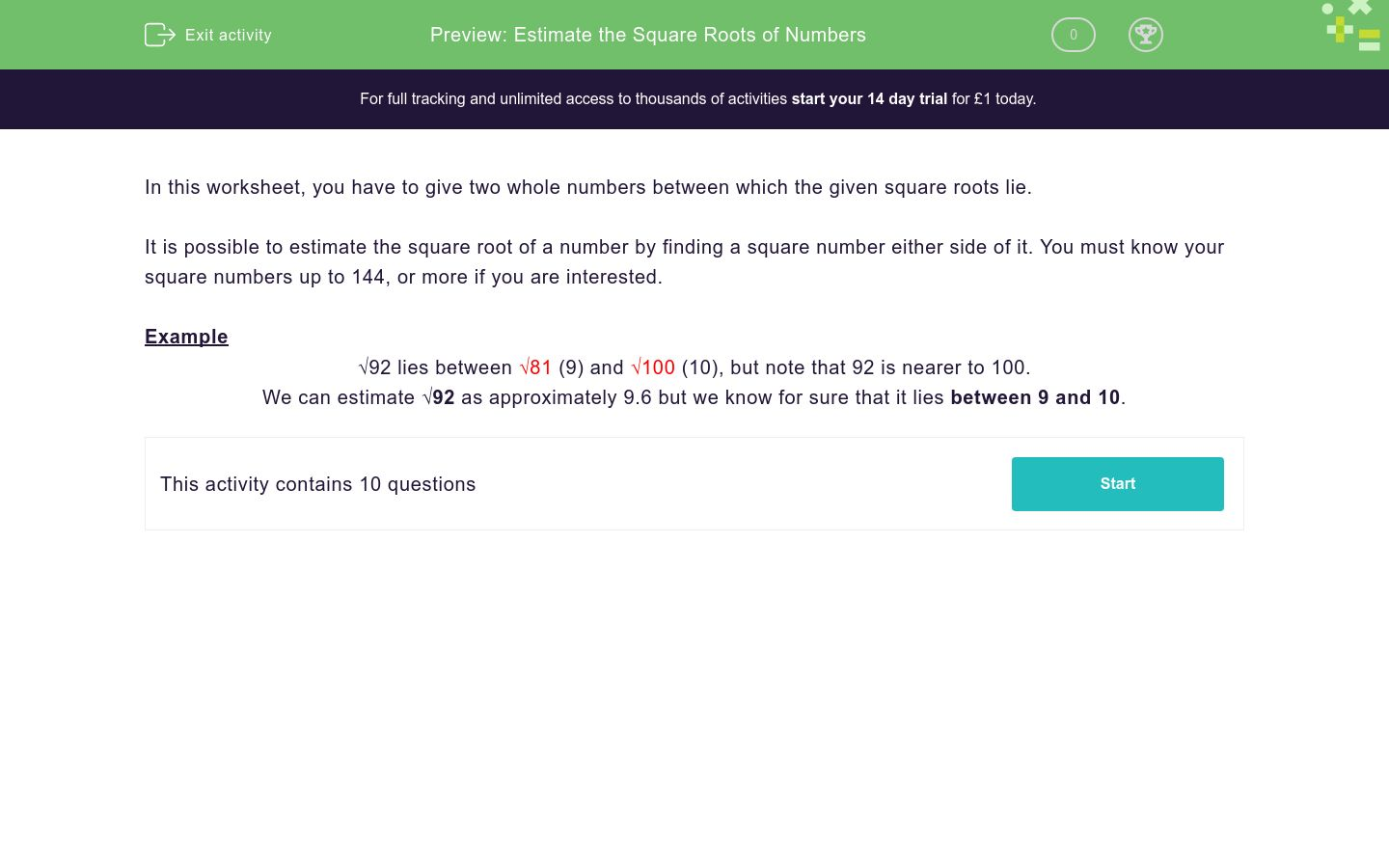# Estimate the Square Roots of Numbers

In this worksheet, students state two numbers between which the given square roots lie.Key stage:  KS 3

Curriculum topic:   Number

Curriculum subtopic:   Understand Order for All Numbers

Difficulty level:### QUESTION 1 of 10

In this worksheet, you have to give two whole numbers between which the given square roots lie.

It is possible to estimate the square root of a number by finding a square number either side of it. You must know your square numbers up to 144, or more if you are interested.

Example

√92 lies between √81 (9) and √100 (10), but note that 92 is nearer to 100.

We can estimate √92 as approximately 9.6 but we know for sure that it lies between 9 and 10.

Estimate the square root of the following number:

√55 lies between _______ & _______

Estimate the square root of the following number:

√37 lies between _______ & _______

Estimate the square root of the following number:

√61 lies between _______ & _______

Estimate the square root of the following number:

√113 lies between _______ & _______

Estimate the square root of the following number:

√62 lies between _______ & _______

Estimate the square root of the following number:

√90 lies between _______ & _______

Estimate the square root of the following number:

√43 lies between _______ & _______

Estimate the square root of the following number:

√26 lies between _______ & _______

Estimate the square root of the following number:

√74 lies between _______ & _______

Estimate the square root of the following number:

√117 lies between _______ & _______

• Question 1

Estimate the square root of the following number:

√55 lies between _______ & _______

7
8
• Question 2

Estimate the square root of the following number:

√37 lies between _______ & _______

6
7
• Question 3

Estimate the square root of the following number:

√61 lies between _______ & _______

7
8
• Question 4

Estimate the square root of the following number:

√113 lies between _______ & _______

10
11
• Question 5

Estimate the square root of the following number:

√62 lies between _______ & _______

7
8
• Question 6

Estimate the square root of the following number:

√90 lies between _______ & _______

9
10
• Question 7

Estimate the square root of the following number:

√43 lies between _______ & _______

6
7
• Question 8

Estimate the square root of the following number:

√26 lies between _______ & _______

5
6
• Question 9

Estimate the square root of the following number:

√74 lies between _______ & _______

8
9
• Question 10

Estimate the square root of the following number:

√117 lies between _______ & _______

10
11
---- OR ----

Sign up for a £1 trial so you can track and measure your child's progress on this activity.

### What is EdPlace?

We're your National Curriculum aligned online education content provider helping each child succeed in English, maths and science from year 1 to GCSE. With an EdPlace account you’ll be able to track and measure progress, helping each child achieve their best. We build confidence and attainment by personalising each child’s learning at a level that suits them.

Get started